## 2.22Region of inﬂuence

A CFD calculation is performed by solving partial diﬀerential equations, such e.g. for conservation of mass, momentum and energy, over a solution domain. It requires suitable boundary conditions, discussed in Chapter 4 .

The solution is inﬂuenced by any change in a ﬁeld value, e.g.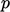,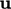,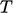, at some point in the domain, e.g. at an inlet boundary. The form of the equation determines the way in which these changes, or perturbations, propagate across the domain over time.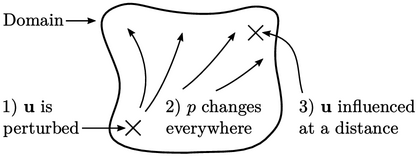Momentum and mass conservation for an incompressible ﬂuid can be represented by Eq. (2.67 ) and Eq. (2.48 ). The form of Eq. (2.48 ), including only a Laplacian derivative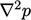, ensures thatis inﬂuenced instantaneously at all points in the domain by a perturbation in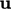at any point.

The resulting instantaneous change in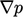then causesto be redistributed everywhere by Eq. (2.67 ), with further short-range changes due to advection and diﬀusion (discussed next).

The outcome for an incompressible ﬂuid is thatis inﬂuenced instantaneously everywhere in the domain by a perturbation at any point. In other words, the speed of sound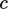, corresponding to propagation of disturbances, is inﬁnite.

Energy conservation can be represented by Eq. (2.65 ), in which perturbations propagate at a characteristic speed due to the advection and diﬀusion.

Advection propagates at speed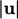, with a region of inﬂuence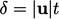in the direction of ﬂow. The relation can be obtained from scale similarity when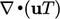and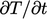are similar in magnitude, i.e.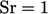in Eq. (2.69 ).

By the same argument, the region of inﬂuence for diﬀusion coincides with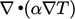and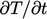being similar in magnitude, i.e.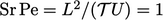. A diﬀusion “front” travels a distance according to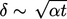(means “of the order of magnitude”).

The diﬀusion equation,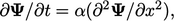in one dimension (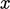) has a solution24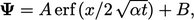where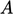,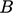are constants and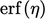is the error function below.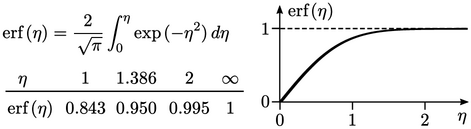This means that for heat conduction problems, e.g. in solids, the distance travelled by the thermal front is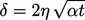, consistent with similarity arguments above.

The coeﬃcient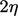depends on where on the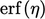curve the front is located. One option is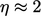, where the solution is within 0.5% of the asymptote of 1.

24Horatio Carslaw and John Jaeger, Conduction of Heat in Solids.

Notes on CFD: General Principles - 2.22 Region of inﬂuence